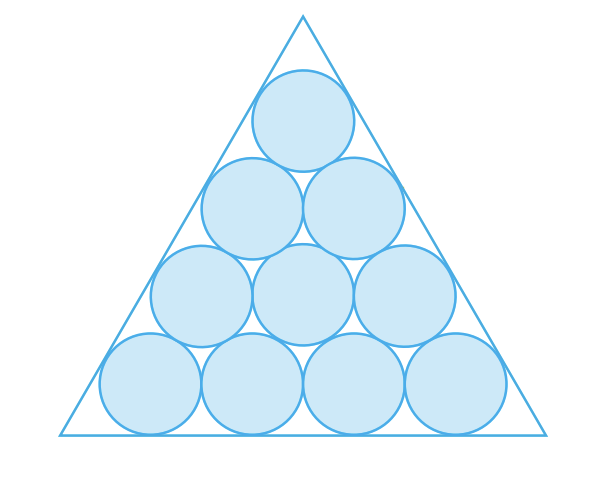# Calculate this awesome limit!

Calculus Level 4Suppose that circles of equal diameter are packed tightly in $n$ rows inside an equilateral triangle. If $A$ is the area of the triangle and $B$ is the total area occupied by the $n$ rows of circles, then find the limit of the ratio $\frac{B}{A}$ (correct to 3 decimal places) as the value of $n$ approaches infinity.

The above figure shows the case for $n = 4$

*Courtesy : Stewart Calculus Early Transcendentals Sixth Edition *

×

Problem Loading...

Note Loading...

Set Loading...